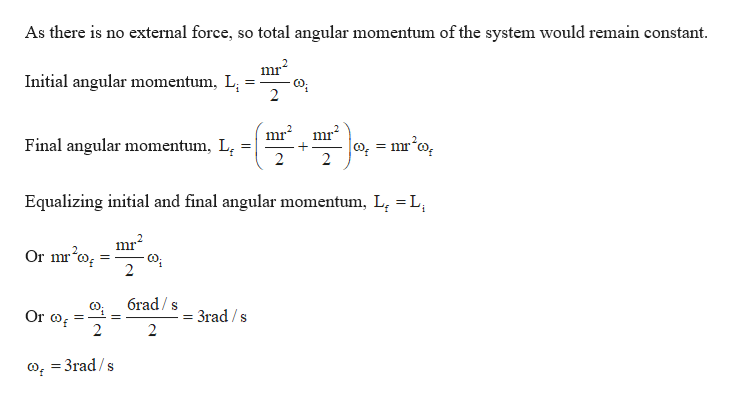# A disk of mass m is spinning freely at 6.00 rad/s when a secondidentical disk, initially not spinning, is dropped onto it so thattheir axes coincide. In a short time the two disks are corotating.(a) What is the angular speed of the new system? (b) If athird such disk is dropped on the first two, find the final angularspeed of the system.

Question
88 views

A disk of mass m is spinning freely at 6.00 rad/s when a second
identical disk, initially not spinning, is dropped onto it so that
their axes coincide. In a short time the two disks are corotating.
(a) What is the angular speed of the new system? (b) If a
third such disk is dropped on the first two, find the final angular
speed of the system.

check_circle

Step 1

Given:

Mass of the disc = m

Step 2

Calculating the new angular velocity if another dis...help_outlineImage TranscriptioncloseAs there is no external force, so total angular momentum of the system would remain constant. Initial angular momentum, L; mr Final angular momentum, L, |0, = mr°o, 2 Equalizing initial and final angular momentum, L, =L, %3D mr Or mro, 6rad / s Or of 3rad / s o, = 3rad /s fullscreen

### Want to see the full answer?

See Solution

#### Want to see this answer and more?

Solutions are written by subject experts who are available 24/7. Questions are typically answered within 1 hour.*

See Solution
*Response times may vary by subject and question.
Tagged in

### Angular Motion